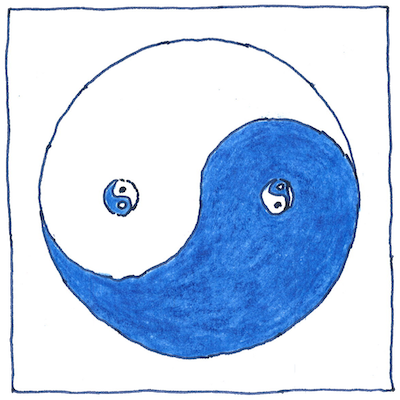# 1679

## The book of science

Tom Sharp

 Gottfried Wilhelm Leibniz mathematics

## Binary number

• Sumerian numbers
• were sexagesimal—base 60.
• Mayan, Aztec, Aruban numbers
• were vigesimal—base 20.
• Egyptian numbers
• were decimal—base 10.
• Yuki and Pamean numbers
• were octal—base 8.
• Numbers in a computer
• are binary—base 2—
• invented by G. W. Leibniz
• in 1679.

## Yin & yang

• Leibniz saw binary numbers
• in the hexagrams of the I Ching.
• Each line of a hexagram
• is either yin or yang.
• With six lines per hexagram
• you get sixth-four hexagrams.
• Leibniz saw in them an affirmation
• of the One and Nothing.

## Location arithmetic

• In 1617 John Napier described
• a non-positional notation
• for binary numbers.
• Instead of two symbols, 0 and 1,
• in Napier’s notation each letter
• of the alphabet represents a power of 2.
• The letter A represents
• 2 to the power of 0, equal to 1.
• The letter B represents
• 2 to the power of 1, equal to 2.
• The letter C represents
• 2 to the power of 2, equal to 4.
• The letter D represents
• 2 to the power of 3, equal to 8.
• To make a number,
• Napier combined letters in any order
• so that DAB, for example equals 11.
• You can replace each letter
• with two of the previous,
• and each doubled letter with the next.
• Subtraction is removal.
• Multiplication by 2
• is replacing with the next.
• Division by 2
• is replacing with the previous.
• All odd numbers contain one A
• or an odd number of A letters.
• *
• In addition to the alphabetic symbols
• for non-positional powers of two,
• Napier proposed an alternative physical device,
• a grid of squares whose coordinates were powers of two.
• Napier’s grid, like other counting tables at the time,
• was similar in principle to the Chinese abacus.
• You could lay out tally markers or jetons
• represent numeric values, add, subtract,
• multiply, divide, and find square roots.

## Cipher

• In 1605 Francis Bacon described a cipher
• for hiding a secret message
• based on two intermixed typefaces
• I learned as a programmer to recognize